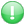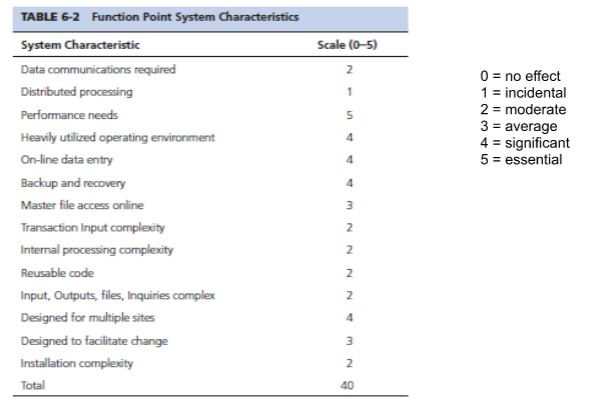Get premium membership and access questions with answers, video lessons as well as revision papers.

# Describe the process of function points

Describe the process of function pointsStep 1: Count the number of business functions within each of the following categories: user inputs, user outputs, inquiries, data structures, and external interfaces
Step 2: Determine the complexity level of each function as simple, medium, or complex. For example output complexity is determined by the number of elements involved, any complex calculations or complex process logic and the number of data structures.
Step 3: Assign weights for each level of complexity within each category
Step 4: Multiply each function by its weight and then sum each product to obtain the total unadjusted function points.
Step 5: Compute the Value Adjustment Factor (VAF)
Step 6: Compute adjusted function point total using the formula
[Unadjusted total x (0.65 + 0.01 x VAF)]Next Step: compute the value adjustment factor based on the following table- Next: calculate the total adjusted function points: Unadjusted total x (0.65 + 0.01 x VAF):
=1050 x (0.65 + 0.01 x 40) = 1102.50
- Results:
Effort = (1.49 hrs effort per f/point) (1102.50) = 1,642.7 hrs
Documentation (.25 pages per f/point) (1102.50) = 275.63 pages
Bugs (.025 bugs per f/point) (1102.50) = 27.5 bugs
User Instructed Rework(.04 per f/point) (1102.50) = 44.1
Dollars ($105.10 per f/point) (1102.50) =$115,872.75
francis1897 answered the question on March 14, 2023 at 06:06

Learn High School English on YouTube